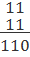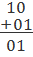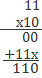## Hexadecimal and Binary Arithmetic

Subject: Computer

#### Overview

The number with base sixteen is called hexadecimal number. We can generate these numbers with the combination of 0, 1, 2, 3, 4, 5, 6, 7, 8, 9, A,B, C, D, E, F. Where A=10, B=11, C=13, D=14, E=15, F=16. This note contains description on hexadecimal number system its conversion and binary arithmetic.
##### Hexadecimal and Binary Arithmetic

The number with base sixteen is called hexadecimal number. We can generate these numbers with the combination of 0, 1, 2, 3, 4, 5, 6, 7, 8, 9, A,B, C, D, E, F. Where A=10, B=11, C=13, D=14, E=15, F=16. We can represent these numbers with suffix sixteen. E.g. (12AB)16 Where A=10, B=11. The 4-bit format of binary is used for hexadecimal to binary conversion.

Weighted value

 Decimal Octal Hexadecimal Binary 0 0 0 0000 1 1 1 0001 2 2 2 0010 3 3 3 0011 4 4 4 0100 5 5 5 0101 6 6 6 0110 7 7 7 0111 8 8 1000 9 9 1001 10 A 1010 11 B 1011 12 C 1100 13 D 1101 14 E 1110 15 F 1111

#### Decimal to Hexadecimal Conversion

The decimal number is repetitively divided by sixteen and remainders are collected to represent hexadecimal numbers.
Example
1. Convert following in hexadecimal number: (1047)10= (417)16

 16 1047 7 16 65 1 4

=(417)16

2. Convert (333)10 into hexadecimal

 16 333 13 16 20 4 4

(333)10= (14D)16 Where D=13

#### Hexadecimal to Decimal

Each hexadecimal digit is multiplied by weighted positions, and sum of product is equal to decimal value.
Example

1. (A37E)16=(?)10
A= 10
E= 14
=Ax163+ 3 x 162+ 7 x 161+ E x 160
=10x163+ 3 x 162+ 7 x 161+ 14 x 160
=40960 + 768 + 112 + 14
(41852)10

#### Binary to Hexadecimal Conversion

The binary numbers are broken into sections of 4-bit digits from last bit and its hexadecimal equivalent is assigned for each section.

Example

1. Convert (11 10 11)2 into base 16.
(11 10 11)2= 11 1011
0011= 3
1011= 11= B
(3B)16
Note: You have to add 00 before first group to make four bits group. (11 to 0011)

#### Hexadecimal to Binary Conversion

Binary equivalent of each hexadecimal digit is written in 4-bit format or section.

Example

Convert following in Binary numbers:

Algorithm

• Convert each Hexadecimal bit into equivalent binary number by making four bits group.
• Arrange all bits to make hexadecimal number.

1. (45AF)16
4= 100= 0100 (Make four digit by adding 0 before the bits)
5= 101= 0101
A=10= 1010
F= 15= 1111
=(010001011010111)2

2. (23AB)16= (0010 0011 1010 1011)2
= 0010, 3= 0011, A= 10, B=11= 1011
=(0010 0011 1010 1011)2

#### Hexadecimal to Octal

• Convert hexadecimal into binary.
• make group bits from last bit.
• convert each into decimal numbers.

Example

1. (ABC)16 to (?)2
(ABC)16
A=10= 1010
B=11= 1011
C= 12= 1100
=(101010111100)2

#### Binary Arithmetic

You have to learn addition, subtraction, multiplication, and division of binary number. In brain, you have to keep that in the arithmetic of binary number, carry is written in binary (2) just like as 10 is used in decimal system for carry.

 Addition Subtraction Multiplication Division 0 + 0 = 0 1 = 0 = 1 0 + 1 = 1 1 + 1 = 0 0 - 0 = 0 1 - 0 = 1 0 - 1 = 1 and carry 1 1 - 1 = 0 0 * 0 = 0 1 * 0 = 0 0 * 1 = 0 1 * 1 = 1 0 · 1 = 0 1 · 0 = not defined 0 · 0 = 0 1 · 1 = 1 ExampleHere, 1+1(right most)= 0 and its carry 1 is added to left columns as 1+1 = 11 Hence, 11+ 11= 110 ExampleHere, 0-1 (right most) = 1 because we take carry 2 from left column and left remains 0. Hence, 10- 01 = 01 ExampleExample1 0 1 0 First number 1 0 0 1 Second number 1 0 0 1 1

Add following binary numbers

1. 1100 + 1111= 11011

 1 Carry 1 1 0 0 1 1 1 1 11 0 1 1

2. 110011+ 111100 + 100110= 10010110

 1 1 1 1 1 Carry 1 1 0 0 1 1 1 1 1 1 0 0 1 0 0 1 1 1 1 0 0 1 0 1 1 0

#### Subtraction

The subtraction of binary is more interesting, but less complex for novice students, but not fear, jump to complement methods when confusing takes place in the traditional methods of subtraction.

Example

110-11=011

The first step is to equalize digits placing zero to the left side and make columns. You take right most columns and solve 0-1.

 1 1 0 0 1 1 1

Next step, come to second column from where you have to solve again 0-1.

 1 1 0 0 1 1 0 1 1

Example

1000-11= 0101

 1 0 0 0 0 1 1 1 0 0 0 1

Example

1000 -1= 111

1000 -10= 110

#### Multiplication

The multiplication of binary number is also like as decimal multiplication.

Example

110 x 11 = 10100

 1 1 0 0 x 1 1 1 1 0 0 1 1 0 0 1 0 0 1 0 0

#### Division

The division process is like as division of decimal numbers.Quotient 1 and remainder 100.

##### Things to remember
• The number with base sixteen is called hexadecimal number.
• Each hexadecimal digit is multiplied by weighted positions, and sum of product is equal to decimal value.
• The decimal number is repetitively divided by sixteen and remainders are collected to represent hexadecimal numbers.
• The binary numbers are broken into sections of 4-bit digits from last bit and its hexadecimal equivalent is assigned for each section.
• Binary equivalent of each hexadecimal digit is written in 4-bit format or section.
• It includes every relationship which established among the people.
• There can be more than one community in a society. Community smaller than society.
• It is a network of social relationships which cannot see or touched.
• common interests and common objectives are not necessary for society.
##### Questions and Answers

A number system that uses sixteen different digits to represent different values is known as hexadecimal number system. The base of hexadecimal number system is 16 because it consist sixteen digits from 0 to 9 and A to F to represent values from ten to fifteen. The digits A, B, C, D, E and F of hexadecimal number represent the decimal numbers 10, 11, 12, 13, 14 and 16 respectively.

The decimal equivalent of a hexadecimal number is the sum of the digits multiplied by 16 with their corresponding weights.

Convert (A2E)16 into Decimal

Solution:

2 1 0 (weight)

Hexadecimal Number: A 2 E

Decimal Equivalent = A×162 + 2×161 + E×160

= 10×256 + 2×16 + 14×1

= 2560 + 32 + 14

= 2606

Hence, (A2E)16 = (2606)10

Hexadecimal digit is represented in 4 bits. A hexadecimal number is converted to its binary equivalent by just substituting the respective binary value for each digit of the hexadecimal number.

Convert (4A5)16 into Binary

Solution:

Hexadecimal Number: 4 A 5

Binary Equivalent: 100 1010 0101 (From Binary Table)

Hence, (4A5)16 = (10010100101)2

There is no any direct method to convert hexadecimal number into octal. So, at first the given hexadecimal number is converted into its binary equivalent then the result will be converted into octal as in previous methods.

Convert (42C)16 into Octal

Solution:

Hexadecimal Number: 4 2 C

Binary Equivalent: 100 0010 1100 (From Binary Table)

Again, Paired Binary

For octal (3 bits): 10 000 101 100 (pairing from right-most digit)

Hexadecimal Equivalent: 2 0 5 4

Hence, (42C)16 = (2054)8

Binary numbers are added to the same manner as decimal numbers. There are only four possible combinations resulting from the addition of two binary digits.

Rules for binary addition:

 A B A+B 0 0 0 0 1 1 1 0 1 1 1 10
i.e. 0 carry 1

The rules for subtraction are the same in the binary system as in the decimal system. The result of the subtraction of binary digit is either 0 or 1.

Rules for binary subtraction:

 A B A-B 0 0 0 1 0 1 1 1 0 0 1 1
i.e. due to borrowing from the next column

Binary numbers are multiplied in the same manner as decimal numbers. We need to remember the rules of binary addition.

Rules for binary multiplication:

 A B A×B 0 0 0 1 0 0 0 1 0 1 1 1

Binary numbers are divided in the same manner as decimal numbers. We need to remember the rules of binary subtraction.

Rules for binary division:

 A B A/B 0 0 0 1 0 1 1 1 Not defined 0 1 Not defined